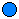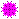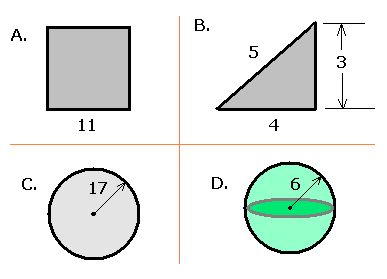### MS 095 PreAlgebra Spring 2003 Q07 • Name: __________

1. _____ What issunimequal to? Marble:Pompom:2. _______________ Give an example of a monomial with a coefficient and a variable raised to the second power.
3. __________ Calculate 110
4. __________ Calculate 10016 ÷ 4
5. __________ Calculate 37 ÷ 0
6. __________ Calculate 0 ÷ 37
7. __________ Calculate 0 ÷ 0
8. __________ Find the square root Ö14641
9. ____________________ Simplify 5x + 7 - 5x2 + 7x - 13 - 2x2:
10. __________ Evaluate 5x + 7 - 5x2 + 7x - 13 - 2x2 for x =3.
11. ____________________ Simplify 5x2 + 3x2
12. ____________________ Multiply ( 5x3y )( 3x2y )
13. ____________________ Multiply ( x - 13 )( 17x + 1 )
14. ____________________ Factor 82362 fully.
15. __________ Find the Greatest Common Factor of 42 and 70
16. __________ Find the Least Common Multiple of 42 and 70
17. __________ Red light plus green light produces what color?
18. For the following diagrams (use p on your calculator or 3.141592 for p:1. __________ What is the perimeter of the square with one side 11 cm in length shown in A?
2. __________ What is the area of the triangle with base 4 cm and height 3 cm shown in B?
3. __________ What is the area of the circle with radius 17 meters shown in C?
4. __________ What is the volume of the sphere with radius 6 meters shown in D?
19. y = _______ Solve -14y = -105 for y.
20. y = _______ Height = 4.48 * t2. If a ball falls for 2.79 seconds, what was the height from which it was dropped?
21. The following adapted data are based on the volumes in cubic centimeters from measurements the class made of the Max® beauty soap boxes:

90, 119, 121, 121, 125, 128, 128, 128

1. ______ What is the mode for the seat count data?
2. ______ What is the median for the seat count data?
3. ______ What is the mean (average) for the seat count data?